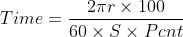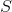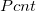# Pivot Full Rotation Time

The time a pivot takes for a 360 degree rotation of a center pivot is often included in the pivot documentation. However, if you don't know this time, use the form below to calculate it. To use the end tower travel speed at the current setting divide the circumference of the last wheel track (2 x pi x radius) and divide by the current travel speed. Learn more about the units used on this page.

Distance From Pivot Point to the Last Tower:
Last Tower Travel Speed at 100% (Minimum full rotation time):
Percentage Timer Setting:
%
Time for 360° (full) Rotation:
Multiply this time by the fraction of a full pivot to get the completion time for wipers. For example for a 1/2 pivot wiper, multiply the above time by 0.5

## The Equation

This calculator uses this formula to determine the time it takes for a center pivot sprinkler to do one full rotation.= Time for full rotation in hours= Distance from pivot point to last tower in ft= Last tower travel speed in ft/min= Pivot percent timer setting

WSU Prosser - IAREC, 24106 N Bunn Rd, Prosser WA 99350-8694, 509-786-2226. Contact Us